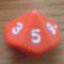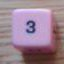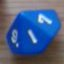Can't Stop

## Can't Stop

 Mon Oct 17 12:31:49 2011   by   Torben Can't Stop (http://boardgamegeek.com/boardgame/41/cant-stop) is a simple board game designed by Sid Sackson.  The board consists of 11 vertical tracks numbered 2 to 12 with between 3 and 13 steps on each: The tracks numbered 2 and 12 each have 3 steps, the tracks numbered 3 and 11 each have 5 steps and so on until the middle track (numbered 7) which has 13 steps.In each round, a player rolls four d6 and combines these into two pairs.  There are three ways of doing this: If the dice are named p, q, r and s, the pairs are {p+q, r+s}, {p+r,q+s} or {p+s,q+r}.  The player chooses one of these pairs and advances a pawn on the two tracks indicated by the sums of the pairs. The player can then choose to roll again and repeat this action or stop.  There is a total of three pawns, so after the second roll, you might not have any pawn to advance because you can't match a pair with a sum equal to one of the tracks that hold pawns.  You are allowed to advance only one pawn if only one pair matches a track with a pawn and there are no unused pawns left, but if you can't match any track, all of your pawns "fall down" to where they were at the start of the player's turn.  If you (before rolling) declare that you stop, you can move a token to where the pawn is and pass the turn to the next player. When a player rolls a pair that matches a track with a token, the pawn starts moving from there.  The first player to get three tokens to the top of their tracks wins.  There are a few other rules, but this is the essence.The name "Can't Stop" is derived from the decision of whether to roll once more and potentially advance the pawns or pass and secure the gains you already have.  Another important decision is which of the three possible pairs to form.  Once you already have played three pawns, the choice is fairly simple: Advancing two pawns is usually better than advancing one, and advancing pawns close to the top is better than advancing pawns near the bottom of their tracks.  But in your first roll, you can normally choose two out of six possible tracks to open.  For this decision, it is good to know which tracks are easiest to complete.  We will try to analyse this below.Naively, you could think that the probability of being able to advance track N is proportional to the probability of rolling N on 2d6.  But this does not take into account the choice of forming pairs of dice. So let us see how we can express this in Troll.  We first roll the four dice:`p := d6; q := d6; r := d6; s := d6;`And then form the six possible pairs:`a := {p+q, r+s, p+r, q+s, p+s, q+r};`To see if this contains an N, we do`N = a`So the total Troll definition is`p := d6; q := d6; r := d6; s := d6;a := {p+q, r+s, p+r, q+s, p+s, q+r};N = a`where N is the sum we want to form.  This gives us the following probabilities for getting at least one way of forming the sum: 2 or 12: 13.194% 3 or 11: 23.302% 4 or 10: 35.751% 5 or  9: 44.753% 6 or  8: 56.096% 7      : 64.352%We say that the "cost" of completing a track is the length of the track divided by the probability of being able to advance the track.  This gives the following costs: 2 or 12: 21.521 3 or 11: 21.457 4 or 10: 19.679 5 or  9: 20.110 6 or  8: 19.609 7      : 20.110So the cheapest tracks are 6 and 8 closely followed by 4 and 10, but the overall differences are fairly minor.When the three pawns are already played, the important question is the probability that you can form one of the three numbers from a roll.  To find this, we can do the following:`p := d6; q := d6; r := d6; s := d6;a := {p+q, r+s, p+r, q+s, p+s, q+r};if {P = a, Q = a, R = a} then 1 else 0`where P, Q and R are the three track numbers.  If the result is 1, it is possible to form at least one of these.There is no room here to list all the 165 possible combinations of P, Q and R, but we can note that the probabilities range from 43.827% for 2, 3, 12 and 2, 11, 12 to 91.975% for 6, 7, 8.  So you should think hard about continuing if your pawns are at the extreme ends but can do so fairly safely if they are near the middle. Mon Nov 14 16:44:28 2011   by   Torben With the new `keep` operator, the line`if {P = a, Q = a, R = a} then 1 else 0`can be replaced by the more readable` if a keep {P,Q,R} then 1 else 0`